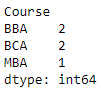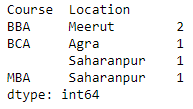# How to count duplicates in Pandas Dataframe?

• Last Updated : 28 Jul, 2020

Let us see how to count duplicates in a Pandas DataFrame. Our task is to count the number of duplicate entries in a single column and multiple columns.

Under a single column : We will be using the `pivot_table()` function to count the duplicates in a single column. The column in which the duplicates are to be found will be passed as the value of the `index` parameter. The value of `aggfunc `will be ‘size’.

 `# importing the module ` `import` `pandas as pd ` `  `  `# creating the DataFrame ` `df ``=` `pd.DataFrame({``'Name'` `: [``'Mukul'``, ``'Rohan'``, ``'Mayank'``,  ` `                             ``'Sundar'``, ``'Aakash'``], ` `                   ``'Course'` `: [``'BCA'``, ``'BBA'``, ``'BCA'``, ``'MBA'``, ``'BBA'``], ` `                   ``'Location'` `: [``'Saharanpur'``, ``'Meerut'``, ``'Agra'``,  ` `                                 ``'Saharanpur'``, ``'Meerut'``]}) ` ` `  `# counting the duplicates ` `dups ``=` `df.pivot_table(index ``=` `[``'Course'``], aggfunc ``=``'size'``) ` ` `  `# displaying the duplicate Series ` `print``(dups) `

Output :Across multiple columns : We will be using the `pivot_table()` function to count the duplicates across multiple columns. The columns in which the duplicates are to be found will be passed as the value of the `index` parameter as a list. The value of `aggfunc `will be ‘size’.

 `# importing the module ` `import` `pandas as pd ` `  `  `# creating the DataFrame ` `df ``=` `pd.DataFrame({``'Name'` `: [``'Mukul'``, ``'Rohan'``, ``'Mayank'``,  ` `                             ``'Sundar'``, ``'Aakash'``], ` `                   ``'Course'` `: [``'BCA'``, ``'BBA'``, ``'BCA'``, ``'MBA'``, ``'BBA'``], ` `                   ``'Location'` `: [``'Saharanpur'``, ``'Meerut'``, ``'Agra'``,  ` `                                 ``'Saharanpur'``, ``'Meerut'``]}) ` ` `  `# counting the duplicates ` `dups ``=` `df.pivot_table(index ``=` `[``'Course'``, ``'Location'``], aggfunc ``=``'size'``) ` ` `  `# displaying the duplicate Series ` `print``(dups) `

OutputMy Personal Notes arrow_drop_up
Recommended Articles
Page :